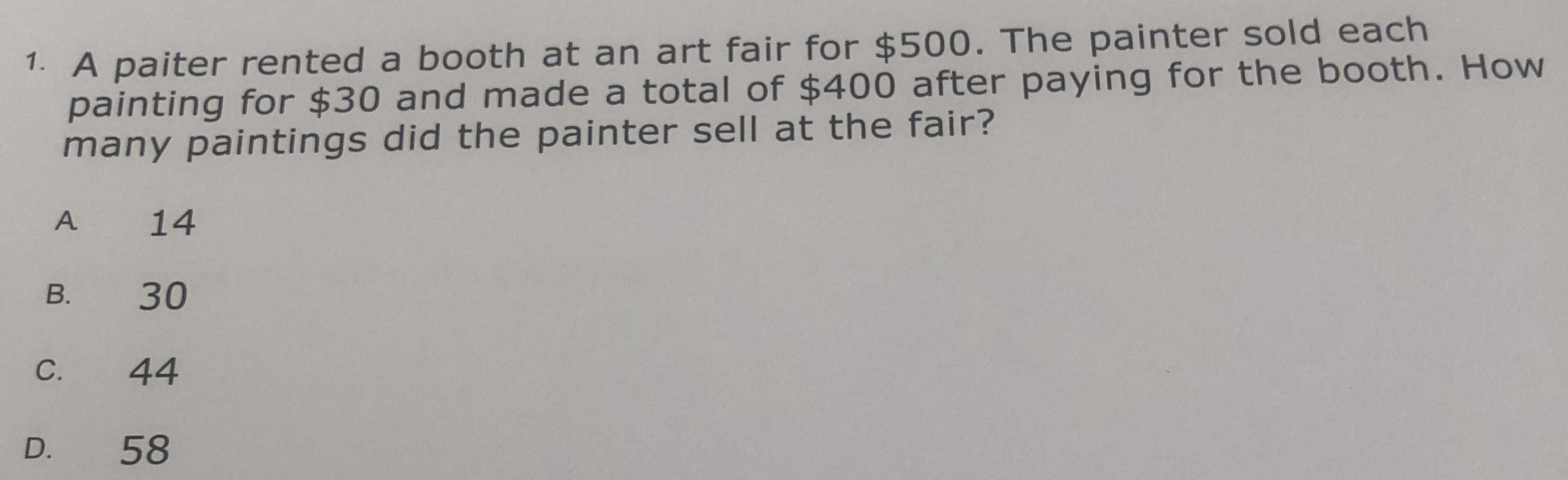### ¿Todavía tienes preguntas de matemáticas?

Pregunte a nuestros tutores expertos
Algebra
Pregunta1. A paiter rented a booth at an art fair for $$\ 500$$ . The painter sold each painting for $$\ 30$$ and made a total of $$\ 400$$ after paying for the booth. How many paintings did the painter sell at the fair?

A. $$14$$ B. $$30$$ C. $$44$$ D. $$58$$